Filters
Latest Questions
CBSE
Mathematics
Lines and angles
What is the method for determining the reference angle for the angle 220?
CBSE
Mathematics
Lines and angles
In figure, $\angle 1 = {60^ \circ }$ and $\angle 6 = {120^ \circ }$. Show that the lines $m$ and $n$ are parallel.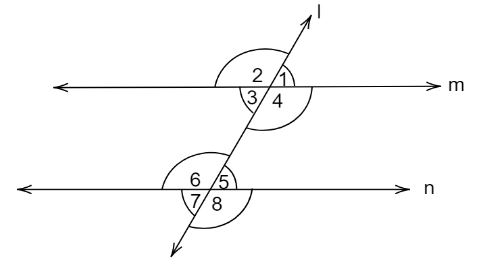CBSE
Mathematics
Lines and angles
Find the value of $x$ in the following figure.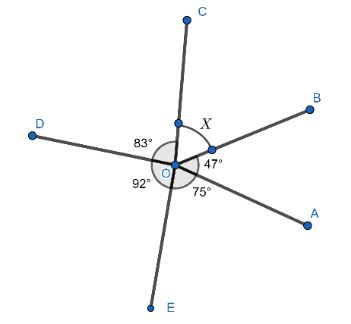CBSE
Mathematics
Lines and angles
Two angles form a linear pair. If the measure of the angles is represented by $3x + 5$ and $x + 15$, then what is the value of x?

CBSE
Mathematics
Lines and angles
Two line segments with common end points form an _____.

CBSE
Mathematics
Lines and angles
In the given diagram , name the point(s):
(a) In the interior of angle $\;DOE$
(b) In the exterior of angle $\;EOF$
(c) On angle $EOF$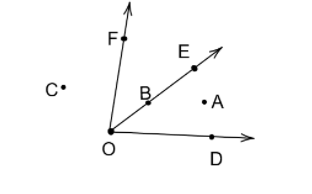CBSE
Mathematics
Lines and angles
Find the number of diagonals of a hexagon.
CBSE
Mathematics
Lines and angles
If one of the angles formed by two intersecting lines is a right angle, what can you say about the other three angles?
a) ${45^ \circ },{45^ \circ },{180^ \circ }$
b) ${90^ \circ },{90^ \circ },{90^ \circ }$
c) ${60^ \circ },{60^ \circ },{90^ \circ }$
d) ${60^ \circ },{90^ \circ },{90^ \circ }$

CBSE
Mathematics
Lines and angles
${L_1}$ and ${L_2}$ are two lines. If the reflection of ${L_1}$ on ${L_2}$ and the reflection of ${L_2}$ is on ${L_1}$ coincides then, the angle between the line is
A. ${30^0}$
B. ${60^0}$
C. ${45^0}$
D. ${90^0}$

CBSE
Mathematics
Lines and angles
Draw a line segment. Take any point C lying in between A and B. Measure the length of AB, BC and AC. Is AB=AC+CB?
[Note: If A,B,C are any three points on a line, such that AC+CB=AB, then we can be sure that C lies between A and B]
CBSE
Mathematics
Lines and angles
Count and name all the angles in these figures: -
(i)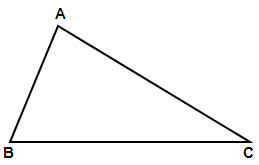(ii)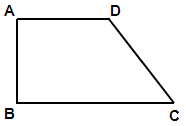CBSE
Mathematics
Lines and angles
Can two angles be supplementary if both of them are?
$\left( i \right)$Acute, $\left( {ii} \right)$Obtuse, $\left( {iii} \right)$Right?

Prev
1
2
3
4
5